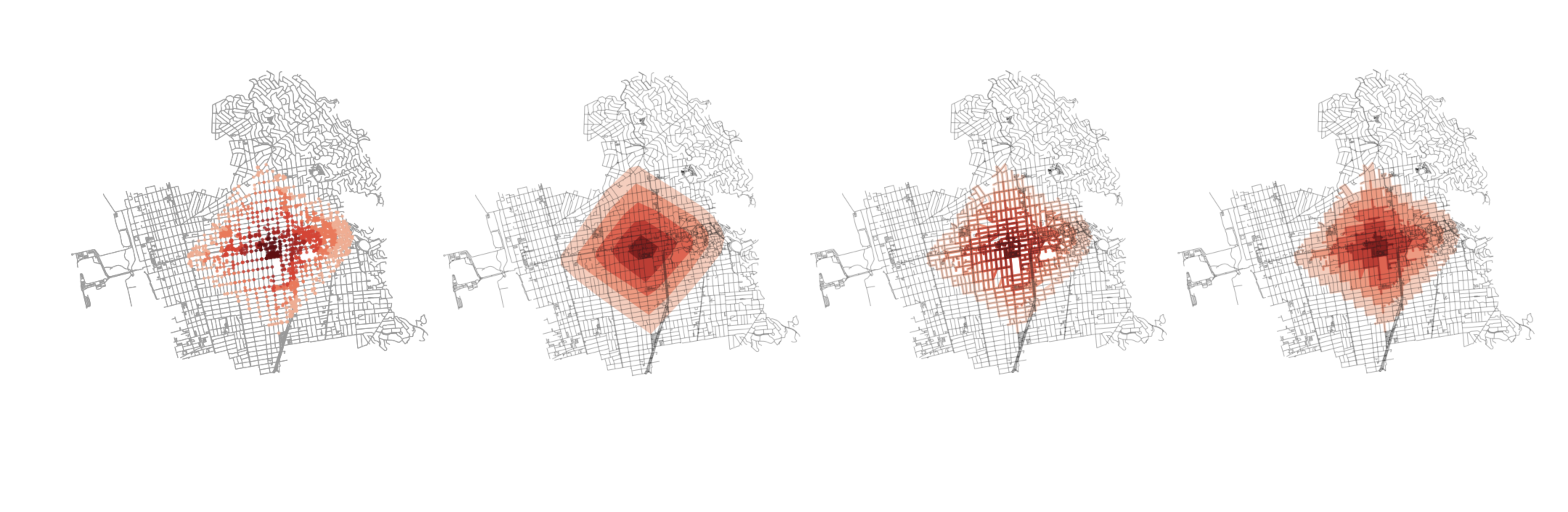# Introduction

The purpose of this post is to document a better method of generating isochrones from a network accessibility operation. OSMnx has a suggested method, outlined here. This method is effective at quickly generating an isochrone, but lacks the ability to effectively render nuanced accessibility patterns as it relies on the generation of a convex hull around the resultant nodes of a given ego graph output.

This is intended to help elaborate on a proposal being submitted under the issues section of the OSMnx examples repo. You can review the proposal here.

### Proposal

I propose a more intensive method that uses both the nodes and edges to create a geometry skeleton of the accessible area. I then allow for a parameterized buffer radius that is then applied to the constructed skeleton. Doing so ensures that a single, complex Polygon object is generated. This prevents downstream issues that would otherwise be caused by merely buffering the nodes, while preserving the nuance that is possible with the buffered nodes option (as opposed to the convex hull geometry).

# Getting started

Here’s all the libraries we will be using to execute this:

Note that this is the same dependencies as in the example script from OSMnx. The only change is that we have added to other Shapely classes, Points and Polygons.

# Review of existing methods

To preserve the contents of that example notebook, should it ever go away, I will include the components here, as well. First load in the example site and set the bucket parameters we will be using.

Now let’s get a point to analyze accessibility from (the center of the project) and also retroject the graph.

It should result in this basic view of Berkeley: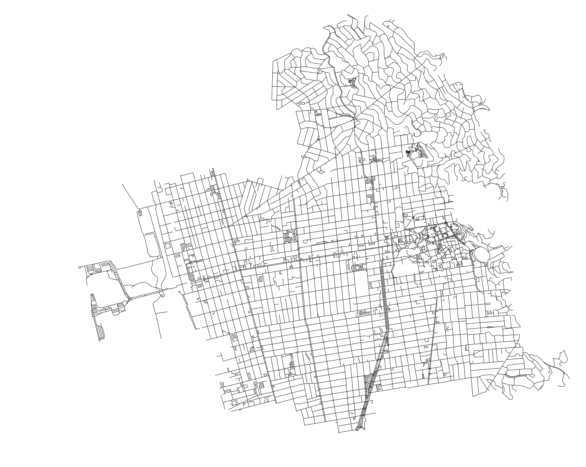Next step (and this is all still just from the notebook) is to add the impedance between edges (the cost to walk from one node to another):

And now generate the iso colors for when we plot access levels:

Let’s run the first version from the notebook, which plots each node and buffers them slightly as well as colors them. This is one way to show accessibility from the original center node:

This should get us to the original dots plot: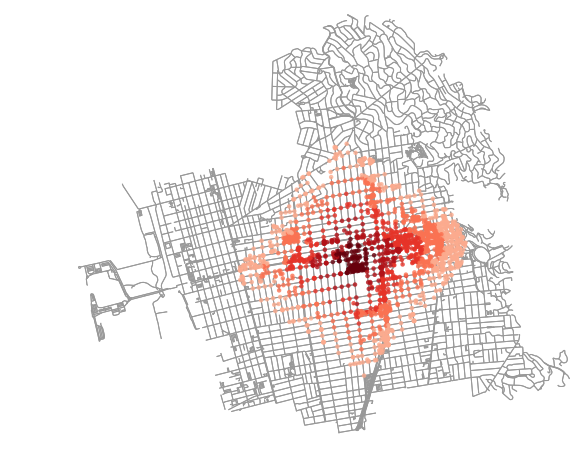Similarly, we can extract those points as point clouds and generate a convex hull around each: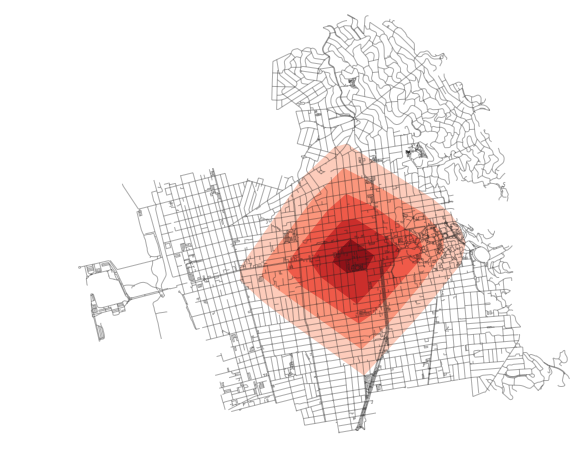There are downsides to both of the above methods. In the first, the dots can be hard to read. Identifying contiguous areas of coverage is tough. Also, downstream, one will have to keep track of the fact that a single coverage area is a MultiPoint or MultiPolygon object and not a single area of coverage which is not intuitive (I would argue).

For the convex hull geometries, all nuance is lost. Imagine if there was a wedge of inaccessible blocks in that area. It would not be covered in the above method. As a result, we would need to devise something that accounts for that and tries to be more nuanced with how it draws coverage area.

# A new method of generating isochrones

I’ll walk through this new method step by step and then wrap it all together at the end. For now, let’s just work with the 25 minute radius. Let’s also set the buffer value to 25 meters (since this is projected in meters). This will be assigned to variable name `buffer_val`.

First, let’s just pull out these nodes and generate that point cloud.

This will generate the following result: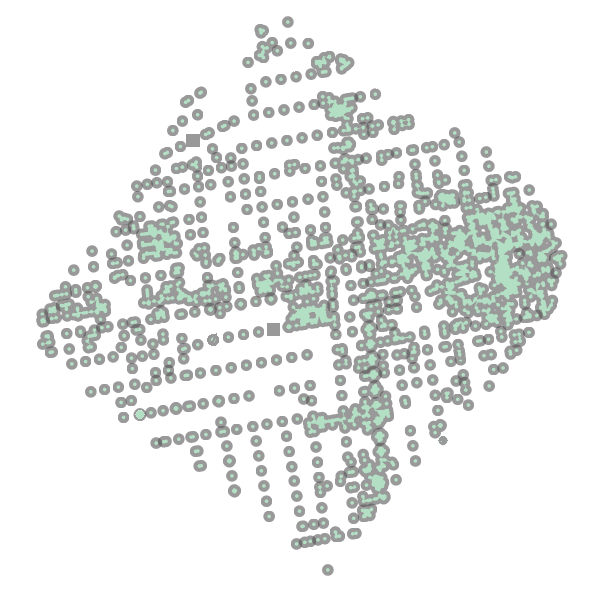Now, let’s do the same for the edges. If we use the edges instead of the nodes, we will get a continuous line set that we can use to plot or, more important, buffer. From this buffer we will achieve on single Polygon representing accessibility at this given time threshold.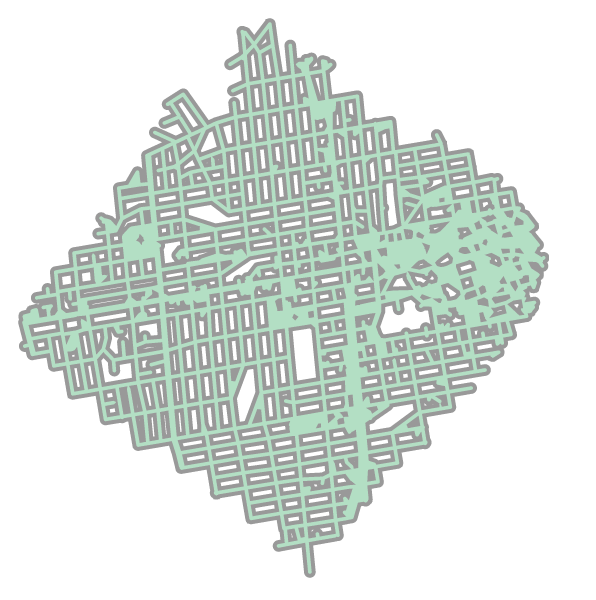Wrapping this all up into a single function, we can write:

This allows us to iterate through each node and each geometry and buffer them a given amount. We can then merge the two together for each threshold isochrone and append them to the list to plot over the network graph, as had previously been done in the OSMnx examples.

Thinner buffer radii will result in a more skeletal isochrone (as shown below):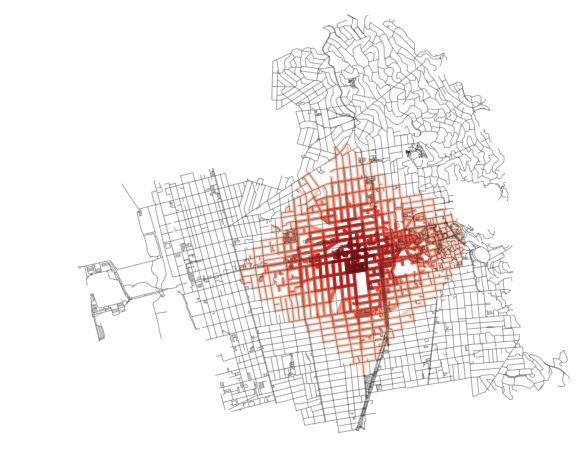Similarly, “thicker” buffers will allow for a more filled in, but also more nuanced, isochrone compared with the convex hull method (as shown below):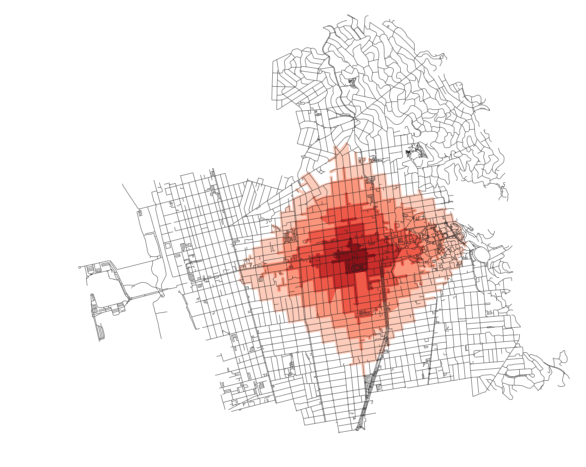Note that in the above, I set `infill` to `True` so that blocks that are surrounded on all sides by edges that are accessible become, themselves, filled in.

And one more, this time with pronounced nodes: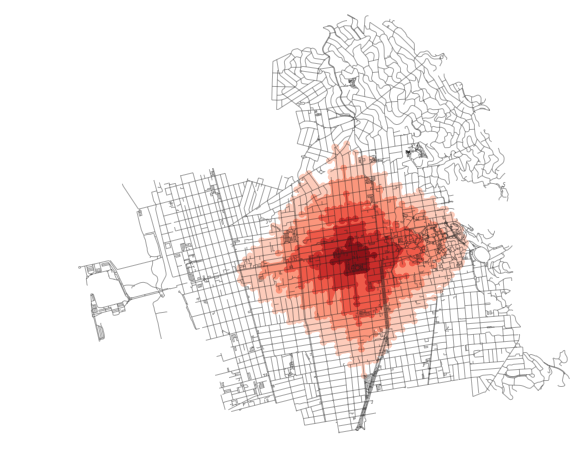Again, each plot above can be produced by simply tweaking the parameters in the `make_iso_polys` method and rerun, like so: